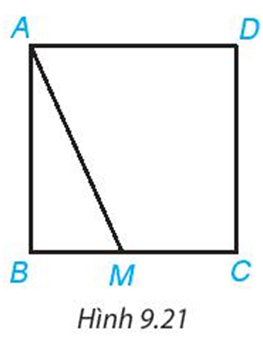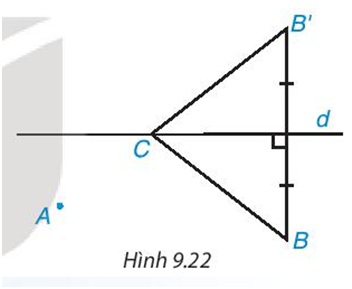## Solve the exercises Joint exercise page 70 (Chapter 9 Math 7 Connect) – Math Book

Solve the exercises Joint practice page 70 (Chapter 9 Math 7 Connect)
============

### Exercise 9.14 page 71 math 7 volume 2 KNTT

Explain: If M is an arbitrary point on the side BC or CD of the square ABCD, the length of the segment AM is always greater than or equal to the length of the side of that square (H.9.21)Solution guide:

• TH1: If M coincides with B. Then AM will coincide with AB and AM = AB
• TH2 : M is a point on BC and does not coincide with B

We have AB ⊥ BC or AB ⊥ BM. So AB is the distance from A to BC, AM is the diagonal from A to bC

=> AB is the shortest path and AM > AB

### Exercise 9.15 page 71 math 7 volume 2 KNTT

Is there a triangle with three sides of length 2.5 cm; 3.4 cm and 6 cm? Why ?

Solution guide:

Considering the triangle inequality, we have: 2.5 + 3.4 = 5.9 < 6.

=> This trio of side lengths cannot form a triangle

Exercise 9.16 page 71 math 7 volume 2 KNTT

Find the perimeter of an isosceles triangle whose sides are 2 cm and 5 cm

Solution guide:

We have a side side of 5 cm, a base side of 2 cm because it satisfies the invariant of the triangle: 5+ 5 > 2 and 5+2 > 5

So the perimeter of an isosceles triangle is: 5.2 + 2= 12 (cm)

### Exercise 9.17, page 71, math 7, volume 2, problem solving skills

The lengths of the two sides of a triangle are 7 cm and 2 cm. Calculate the length of the remaining side knowing that its measure in centimeters is an odd natural number

Solution guide:

Let the length of that side be X

According to the triangle inequality, we have: X < 2+ 7 => X < 9

7 < X + 2 => X > 5

So 5 < X < 9. We have the following numbers: 6,7,8

According to the problem, the measure is an odd natural number. So X = 7. The length needed is 7 cm

### Exercise 9.18 page 71 math 7 volume 2 KNTT

Know two sides of a triangle of length a and b. Based on the triangle inequality, explain why the perimeter of the triangle is greater than 2a and less than 2 (a+b).

Solution guide:

Let the length of the remaining side be c. P is the perimeter of the triangle

According to the triangle inequality, we have a < b + c

=> a+ a < a+ b+ c

=> 2a < P

According to the triangle inequality, we have: a+ b > c

=> a + b + a > a+c

=> 2a + b > a+ c

=> 2a + b + b > a + c + b

=> 2a + 2b > a+c +b

=> 2(a+b) > P

So we have the conclusion 2a < P < 2( a+b)

### Exercise 9.19 page 71 math 7 volume 2 KNTT

Two gardens A and B are on one side of the canal d. Determine the side of the canal on the same side A and B, a point C to place the water pump from the irrigation canal for the two gardens so that the total length of the pipeline leading from the pump to the garden is the shortest (HD: Call B’ is a point such that d is the perpendicular bisector of BB’ (H.9.22), then CB = CB’.Solution guide:

We have: AC + BC AB (because C is an undefined point)

Therefore: AC + BC is shortest when AC + BC = AB

=> A, B, C align and C lies between A; B.

So the position to put the water pump from the irrigation canal for the two gardens so that the shortest total length of water pipe is C between A and B (and A, B, C are aligned)